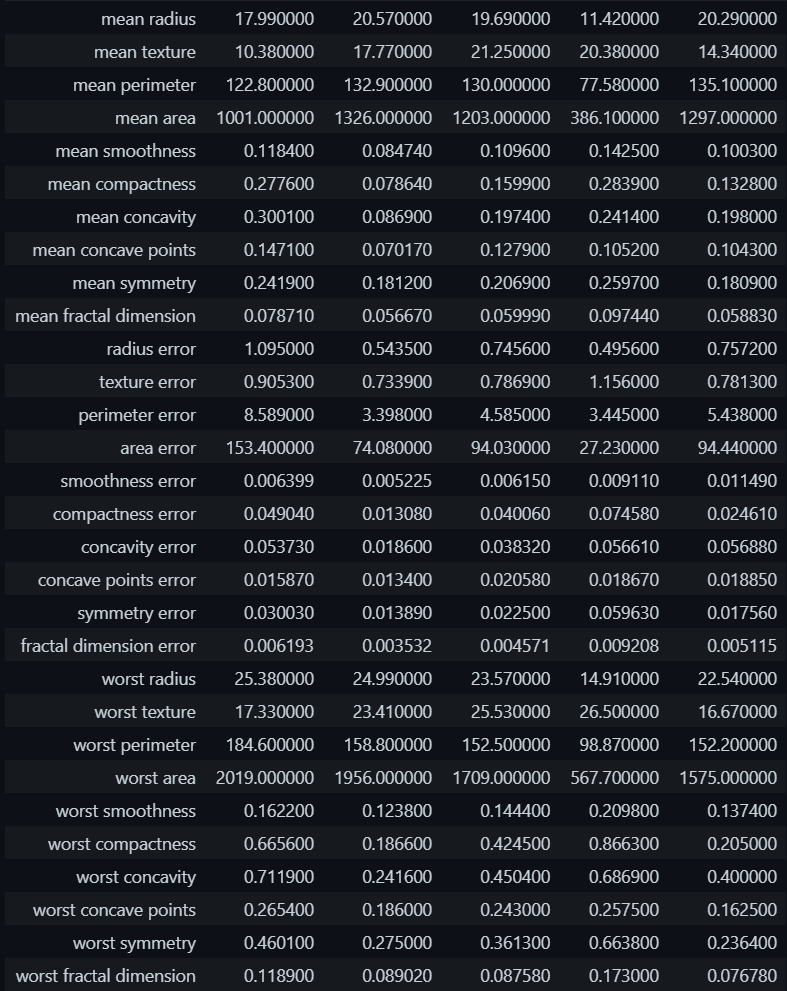Open In App

# Python Sklearn – sklearn.datasets.load_breast_cancer() Function

In this article, we are going to see how to convert sklearn dataset to a pandas dataframe in Python.

Sklearn is a python library that is used widely for data science and machine learning operations. Sklearn library provides a vast list of tools and functions to train machine learning models.

The library is available via pip install.

`pip install scikit-learn`

There are several sample datasets present in the sklearn library to illustrate the usage of the various algorithms that can be implemented through the library. Following is the list of the sample dataset available –

It is used to load the breast_cancer dataset from Sklearn datasets.

Each of these libraries can be imported from the sklearn.datasets module. As you can see in the above datasets, the first dataset is breast cancer data. We can load this dataset using the following code.

## Python3

 `from` `sklearn.datasets ``import` `load_breast_cancer``data ``=` `load_breast_cancer()`

The data variable is a custom data type of sklearn.Bunch which is inherited from the dict data type in python. This data variable is having attributes that define the different aspects of dataset as mentioned below.

The following code produces a sample of the data from the breast cancer dataset.

## Python3

 `import` `pandas as pd``data_df ``=` `pd.DataFrame(data ``=` `data.data,``                       ``columns ``=` `data.feature_names)``data_df.head().T`

Output:Sample Data Records – Breast Cancer Dataset